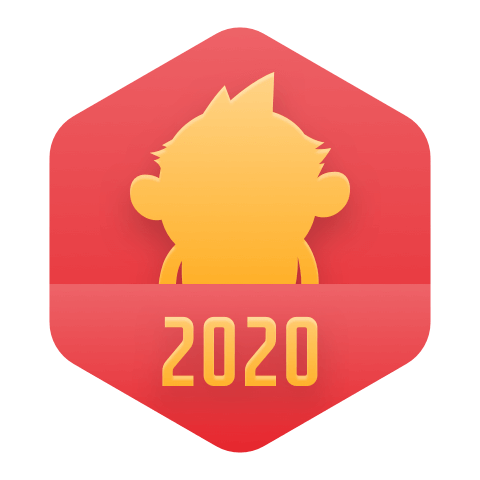### Neural Network Programming with Java_code下载GitHubGitHub[资源帖]ICML2016 下载地址

2008最新2000多本最有价值的程序设计电子教程下载

A B C D E F G H I J K L M N O P Q R S T U V W X Y Z OT A 回顶部A Computational Differential Geometry Approach to Grid Generation Second E

Neural Network Programming with Java_code

Neural Network Programming with Java

Neural.Network.Programming.with.Java.17858809

One of the most preferred languages for neural network programming is Java as it is easier to write code using it, and most of the most popular neural network packages around already exist for Java....

Hands-On Network Programming with C# and .NET Core.epub

Hands-On Network Programming with C# and .NET Core: A comprehensive guide to understanding network architecture, communication protocols, and network analysis to build secure applications compatible ...

Logistic Regression with a Neural Network mindset 参考答案

Logistic Regression with a Neural Network mindsetWelcome to your first (required) programming assignment! You will build a logistic regression classifier to recognize cats. This assignment will step y

Deep Learning: Practical Neural Networks with Java 完整高清英文azw3版

Neural Network Programming with Java, Second Edition Style and approach This course aims to create a smooth learning path that will teach you how to effectively use deep learning with Java with ...

Neural+machine+translation+with+attention+-+v3

Neural Machine ...Welcome to your first programming assignment for this week! You will build a Neural Machine Translation (NMT) model to translate human readable dates (“25th of June, 2009...

Deep Neural Network for Image Classification: Application When you finish this, you will have finished the last programming assignment of Week 4, and also the last programming assignment of this cours

big code: Code Completion for Programming Education based on Recurrent Neural Network [IWCIA 2019]

Python Basics with numpy (optional)Welcome to your first (Optional) programming exercise of the deep learning specialization. In this assignment you will: - Learn how to use numpy. - Implement some ...

Logistic Regression with a Neural Network mindset v4 课程一第二周编程作业

Logistic Regression with a Neural Network mindsetWelcome to your first (required) programming assignment! You will build a logistic regression classifier to recognize cats. This assignment will step y

DL-1-week2-Basics of Neural Network Programming

2 Basics of Neural Network Programming 2.1 Binary Classification 2.2 Logistic Regression 2.3 Logistic Regression cost function 2.4 Gradient Descent 2.5 Derivatives 2.6 More derivatives examples 2.7 .....

Art: Neural Style Transfer Welcome to the second assignment of this week. In this assignment, you will learn about Neural Style Transfer. This algorithm was created by Gatys et...

[coursera/dl&nn/week2]Basics of Neural Network programming(quiz)

This blog helps me review the course on coursera. Wrong answer: 3.reshape to a column vector 9."*" means the elementwise product  ".dot" means matrix multiplication operation ...

Pytorch——PyTorch Tensors Explained - Neural Network Programming

Pytorch——Deeplizard视频学习（Part2）PyTorch Tensors Explained - Neural Network ProgrammingInstances of the *torch.Tensor* classTensor attributesTensors have a *torch.dtype*Tensors have a *torch....

[coursera/dl&nn/week2]Basics of Neural Network programming(2.2 py & Vectorization)

2.2 Python and Vectorization 2.2.1 Vectorization  We use a loop to iterate the training data, it costs long when meets a large ...Vectorization is the secret why numpy have pri

Art Generation with Neural Style Transfer - v2

Deep Learning & Art: Neural Style TransferWelcome to the second assignment of this week. In this assignment, you will learn about Neural Style Transfer. This algorithm was created by Gatys et al. ...

Logistic Regression with a Neural Network mindset Welcome to the first (required) programming exercise of the deep learning specialization. In this notebook you will build your first image recogn...

Logistic Regression with a Neural Network mindset Welcome to your first (required) programming assignment! You will build a logistic regression classifier to recognize cats. This assignment will step

Neural Networks and Deep Learning--Course1week4--Deep Neural Network - Application v8

Deep Neural Network for Image Classification: Application When you finish this, you will have finished the last programming assignment of Week 4, and also the last programming assignment of this cours...

Art Generation with Neural Style Transfer-v2

neural networks deep learning Logistic Regression with a Neural Network mindset Homework

coursera neural networks deep learning编程参考答案

make your own neural network tariq rashid

We introduce the popular and easy to learn Python programming language, and gradually builds up a neural network which can learn to recognise human handwritten numbers, easily getting it to perform ...

Logistic Regression with a Neural Network mindset v3

Logistic Regression with a Neural Network mindset¶ Welcome to your first (required) programming assignment! You will build a logistic regression classifier to recognize cats. This assignment will ste

Deep Neural Network for Image Classification: Application When you finish this, you will have finished the last programming assignment of Week 4, and also the last programming assignment of this cour

Deep Neural Network - Application v3

Deep Neural Network for Image Classification: ApplicationWhen you finish this, you will have finished the last programming assignment of Week 4, and also the last programming assignment of this course...

Machine Learning week 5 programming exercise Neural Network Learning

Neural Networks Learning  这次试用的数据和上次是一样的数据。5000个training example，每一个代表一个数字的图像，图像是20x20的灰度图，400个像素的每个位置的灰度值组成了一个training example。